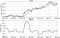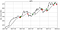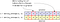# Analyzing Stock Price Time Series with Fortran Arrays, Part 3

From Modern Fortran by Milan Curcic

__________________________________________________________________

Take 37% off Modern Fortran. Just enter code fcccurcic into the discount box at checkout at manning.com.
__________________________________________________________________

In this part, we’ll conclude the article by using built-in functions and whole-array arithmetic to identify risky stocks, and find good times to buy and sell.

Part 3: Identifying risky stocks and when to buy and sell

In Part 1, I explained how to declare and initialize dynamic Fortran arrays. I also showed how to use the so-called array constructors to conveniently allocate and initialize an array in a single line of code. In Part 2, we went into more detail on explicit allocation and deallocation of Fortran arrays, which gives you finer control such as using custom index ranges for your arrays. Explicit allocation also comes with built-in exception handling, allowing you to write more robust and fault-tolerant code. You also learned how to slice arrays with arbitrary range and stride. If you haven’t yet read the first two parts, I recommend you go back and do that before moving on. In the third and final part, we’ll use built-in functions and whole-array arithmetic to calculate some interesting metrics such as moving average and standard deviation. We’ll use these to tackle the remaining two stock price analysis challenges — identifying risky stocks, and finding good times to buy and sell.

One of the ways to estimate how risky is a stock at some time is by looking at the so-called volatility. Stock volatility can be quantified as the standard deviation of the stock price relative to its average. Standard deviation is a statistical measure that tells you how much individual array elements deviate from the average. To estimate volatility, we’ll implement functions to compute average and standard deviation given an arbitrary input array. Furthermore, we’ll compute these metrics over a limited time window, and sliding that window along the whole time series. This is the so-called moving average. For example, Figure 3 shows the actual price, 30-day moving average, and volatility based on 30-day moving standard deviation for Nvidia stock.Figure 1: Nvidia stock price (black) and a 30-day simple moving average (grey). Bottom: Volatility expressed as standard deviation relative to the 30-day simple moving average, in percent.

While the Fortran standard library comes with many mathematical functions out of the box, it doesn’t include a function to compute the average of an array. However, it is fairly straightforward to implement using intrinsic functions `sum` and `size`:

`pure real function average(x)      real, intent(in) :: x(:)      average = sum(x) / size(x)  end function average`

We already saw earlier that we can use `size` in a `do`-loop when we want to iterate over all elements of an array, or when we want to reference the last element of an array. `sum` does exactly what you think it does – you pass it an array and it returns the sum of all elements.

To calculate the standard deviation of an array `x`, we can follow these steps:

1. Calculate the average of the array. For this, we can use the function that we just wrote: `average(x)`. The result is a scalar (non-array).
2. Find the difference between each element of the array and its average. This is where the power of whole-array arithmetic comes in. We can use the subtraction operator `-` that we are familiar with, and we can apply it directly on the whole array, without the need for looping: `x - average(x)`. When applying arithmetic operators (`+`, -, `*`, `/`, `**`), assignment (`=`), or comparison (`>`, `>=`, `<=`, `<`, `==`, `/=`) operators, they will be applied to each element individually. In this case, `x` is an array and `average(x)` is a scalar, `x - average(x)` will subtract `average(x)` from each element of `x`. The result is an array.
3. \Square the differences. Same as in step 2, except this time we need to take the power of 2: `(x - average(x))**2`. In this expression, `**` is the power operator.
4. Calculate the average of the squared differences. We can apply the same function again: `average((x - average(x))**2)`.
5. And finally, take the square root of the result from step 4. For this, we can use the intrinsic `sqrt` function, which is also an elemental function – it works on both scalars and arrays.

Here’s the complete code for the standard deviation function. Thanks to the Fortran whole-array arithmetic, we can express all 5 steps as a one-liner:

`pure real function std(x)      real, intent(in) :: x(:)      std = sqrt(average((x - average(x))**2))  end function std`

To use arithmetic operators on whole arrays, the arrays on either side of the operator must be of the same shape and size. You can also combine an array of any shape and size with a scalar. For example, if `a` is an array, `2 * a` will be applied element-wise, that is, each element of `a` will be doubled.

Style Tip
Use whole-array arithmetic over `do`-loops whenever possible.

However, we are not done here. Rather than just the average and standard deviation of the whole time series, we are curious about these metrics that are relevant to a specific time, and we want to be able to see how they evolve. For this we can use the average and standard deviation along a moving time window. A commonly used metric in finance is the so-called simple moving average, which takes an average of some number of previous points, moving in time. I will let you tackle this one, and will meet you on the other side of this exercise.

Our current implementations for average and standard deviation are great but they don’t let us specify a narrow time period that would give us more useful information about stock volatility at a certain time. Let’s write a function `moving_average` that takes an input real array `x` and integer window `w`, and returns an array that has the same size as `x`, but with each element being an average of `w` previous elements of `x`. For example, for `w = 10`, the moving average at element `i` would be the average of `x(i-10:i)`. In finance, this is often referred to as the simple moving average.

We can use the intrinsic function `max` to limit the indices near the edges of `x` to prevent going out of bounds. For example, `max(i, 1)` results in `i` if greater than 1, and 1 otherwise. We’ll also need to use a combination of looping and whole-array arithmetic to implement the solution.

The moving average can be implemented by iterating over each element of the input array, slicing that array over a sub-range determined by the input window parameter, and applying the general average function to that slice:

`pure function moving_average(x, w) result(res)      real, intent(in) :: x(:)      integer, intent(in) :: w      real :: res(size(x)) ! declare res with same size as x      integer :: i, i1      do i = 1, size(x)          i1 = max(i-w, 1) ! ensure i1 is never < 1          res(i) = average(x(i1:i)) ! average over the window      end do  end function moving_average`

Notice that inside the loop, I use the intrinsic functions `min` and `max` to limit the sub-range from exceeding the bounds of the array `x`. For a standard deviation function over an equivalent window, we’d just replace `average(x(i1:i))` with `std(x(i1:i))`. You can find these functions in `stock-prices/src/mod_arrays.f90`.

The main program of this challenge is very similar to the previous one (`stock_gain`). However, besides printing the total time series average and volatility on the screen, now we also write the 30-day moving average and standard deviation into text files:

`program stock_volatility     use mod_arrays, only: average, std, moving_average,&                         moving_std, reverse   use mod_io, only: read_stock, write_stock   ...   do n = 1, size(symbols)     ...     im = size(time)     adjclose = reverse(adjclose)     ...     call write_stock(trim(symbols(n)) // '_volatility.txt',&       time(im:1:-1), adjclose,&       moving_average(adjclose, 30), moving_std(adjclose, 30))     end do   end program stock_volatility`

Look inside `stock-prices/src/mod_io.f90` to see how is the `write_stock` subroutine implemented. The full program is located in `stock-prices/src/stock_volatility.f90.`

Can we use historical stock market data to learn some techniques that could help us determine a good time to buy or sell a certain stock? One of the commonly used indicators by traders is the so-called moving average crossover. Consider that the simple moving average is general indicator of whether the stock is going up or down. For example, a 30-day simple moving average would tell you about the overall stock price trend. You can think of it of a smoother and delayed stock price, without the high frequency fluctuations. Combined with the actual stock price, we can use this information to decide whether we should buy or sell, or do nothing — see Figure 4.Figure 2: Moving average crossover indicators for Apple. Black is the adjusted daily closing price, grey is the 30-day simple moving average, and up and down arrows are the positive and negative crossover markers, respectively.

In this figure, I marked with an up arrow every point in time that the actual price crossed the moving average line from low-to-high, and with a down arrow when crossing from high-to-low. The rule of thumb is: sell at the point when the actual price drops below the moving average line, and buy at the point when it rises above the moving average line.

Let’s see how we can employ Fortran arrays and arithmetic to compute the moving average crossover. The calculation will consist of two steps:

Compute the moving average over some time period. It can be any period of time, depending on the trends that you are interested in (intra-day, short term, long term, etc.), and the frequency of the data that you have. We are working with daily data so we’ll work with a 30-day moving average. We’ve already implemented the `moving_average` function in the previous section, and you can find it in `stock-prices/src/mod_arrays.f90`.

Once we have the moving average, we can follow the actual stock price and find times when it crosses the moving average line. If the actual price crosses the moving average from below going up, it’s an indicator of potentially good time to buy. Otherwise, if it crosses from above going down, it’s likely a good time to sell.

The main trick we’ll use for this challenge is to determine all array indices where the stock price is greater than its moving average, and also those where the stock price is smaller than its moving average. We can than combine these two conditions, and find all the indices where the stock price changes from smaller to greater, and vice versa:Figure 3: Finding indices where the stock price crosses its moving average.

To implement this calculation, we’ll use almost all array features that we have learned about in this article so far: assumed-size and dynamic array declaration, array constructor, and invoking a custom array function (`moving_average`). Further, we’ll also create logical (Boolean) arrays to handle the conditions I described in Figure 5. Finally we’ll employ an intrinsic function `pack` to select only those indices that satisfy our criteria.

All this can be written in about a dozen lines of code:

`pure function crosspos(x, w) result(res)  real, intent(in) :: x(:)  integer, intent(in) :: w  integer, allocatable :: res(:)  real, allocatable :: xavg(:)  logical, allocatable :: greater(:), smaller(:)  integer :: i  res = [(i, i = 2, size(x))] ! initialize function result  xavg = moving_average(x, w)  greater = x > xavg ! True where x is greater than xavg  smaller = x < xavg ! True where x is smaller than xavg  res = pack(res, greater(2:) .and. smaller(:size(x-1)))end function crosspos`

We first initialize our result array `res` as an integer sequence from 2 to `size(x)`. This is our first guess from which we’ll subset only those elements that satisfy our criteria. The crux is in the last executable line where we invoke the `pack` function. How does `pack` work? When you have an array `x` that you want to subset according to some condition `mask`, invoking `pack(x, mask)` will as a result return only those elements of `x` where `mask` is true. `mask` doesn’t have to be a logical array variable – it can be an expression as well, which is how we used it in our function above. Recall the automatic re-allocation on assignment from Part 2? This is exactly where it comes in super handy – we pass the original array `res` and a conditional mask to `pack()`, and it returns a smaller, re-allocated array `res` according to the mask.

This function only returns the crossover from low to high value, thus named `crosspos`. However, we also need the crossover from high to low, so that we know when the stock price is going to drop below the moving average curve. How would we implement the negative variant of the crossover function, `crossneg`? We can reuse all the code from `crosspos`, except for the last criterion – we need to look for elements that are going from higher to lower, instead of lower to higher:

`pure function crossneg(x, w) result(res)  ...  res = pack(res, smaller(2:) .and. greater(:size(x-1)))end function crossneg`

The main program will use these functions to find the indices from the time series, and write the matching timestamps into files:

`program stock_crossover  ...  use mod_arrays, only: crossneg, crosspos, reverse  ...  integer, allocatable :: buy(:), sell(:)  ...  do n = 1, size(symbols)    ...    time = time(size(time):1:-1)    adjclose = reverse(adjclose)     buy = crosspos(adjclose, 30)    sell = crossneg(adjclose, 30)      open(newunit=fileunit, file=trim(symbols(n)) // '_crossover.txt')    do i = 1, size(buy)      write(fileunit, fmt=*) 'Buy ', time(buy(i))    end do    do i = 1, size(sell)      write(fileunit, fmt=*) 'Sell ', time(sell(i))    end do    close(fileunit)   end do  end program stock_crossover`

I have included only the relevant new code in this listing. The full program is located in `stock-prices/src/stock_crossover.f90`. The program itself doesn’t do much new stuff. For each stock, it invokes the moving average crossover functions and stores the results into arrays (`buy` and `sell`), and writes the timestamps with these indices into a text file. I have plotted the results for Apple (AAPL) for 2017 in Figure 5. You can use the included Python scripts to plot the results for other stocks and other times.

Plotting the results
Both the second and third challenge in this article produce results that I plotted and showed above. The Python plotting scripts that I used are included in the `stock-prices/plotting`directory. Follow the directions in `README.md` to set up your own Python plotting environment. If you decide to further explore the stock prices data, you can use and modify these scripts for your own application.

And that’s it, we’ve made it! Following only a few rules for array declaration, initialization, indexing, and slicing, we have written a nifty little stock analysis app that tells us some quite useful things about the longer term trends and risk of individual stock prices. The skills that you’ve learned in this article form a foundation for learning parallel Fortran programming with coarrays.

1. Declare, allocate, and initialize arrays.
2. Index and slice arrays to reference specific elements.
3. Use whole-array operators and arithmetic for stock price analysis.

I covered some of the most powerful features of Fortran arrays. First, we went over the detailed workings of allocating and deallocating dynamic arrays. The exception handling that comes built into these features will help you write more robust and fault-tolerant apps. We then covered all the ways to index and slice arrays to select only those elements that we need. Slicing in particular showed to be useful when computing the moving average for estimating stock volatility and finding good times to buy or sell shares of a stock. However, always be careful not to go out-of-bounds when referencing Fortran arrays! This can often go unnoticed by the compiler, and can result in those pesky segmentation faults that are so difficult to debug, or worse, corrupted data without warning.

You may have noticed that even though the data we used in this article had several different variables, including daily high and low prices and number of shares traded, I only used the time stamps and the adjusted close price. For our exercise that was enough, but if you’re interested in doing more, I encourage you to explore other variables in the dataset. The `read_stock` subroutine is already written to parse all the other variables, so you can take it from there. Let me know if you expand this into a more full-fledged stock analysis app – I would love to hear more about it!

If you want to learn more about the book, check it out on liveBook here and see this slide deck.